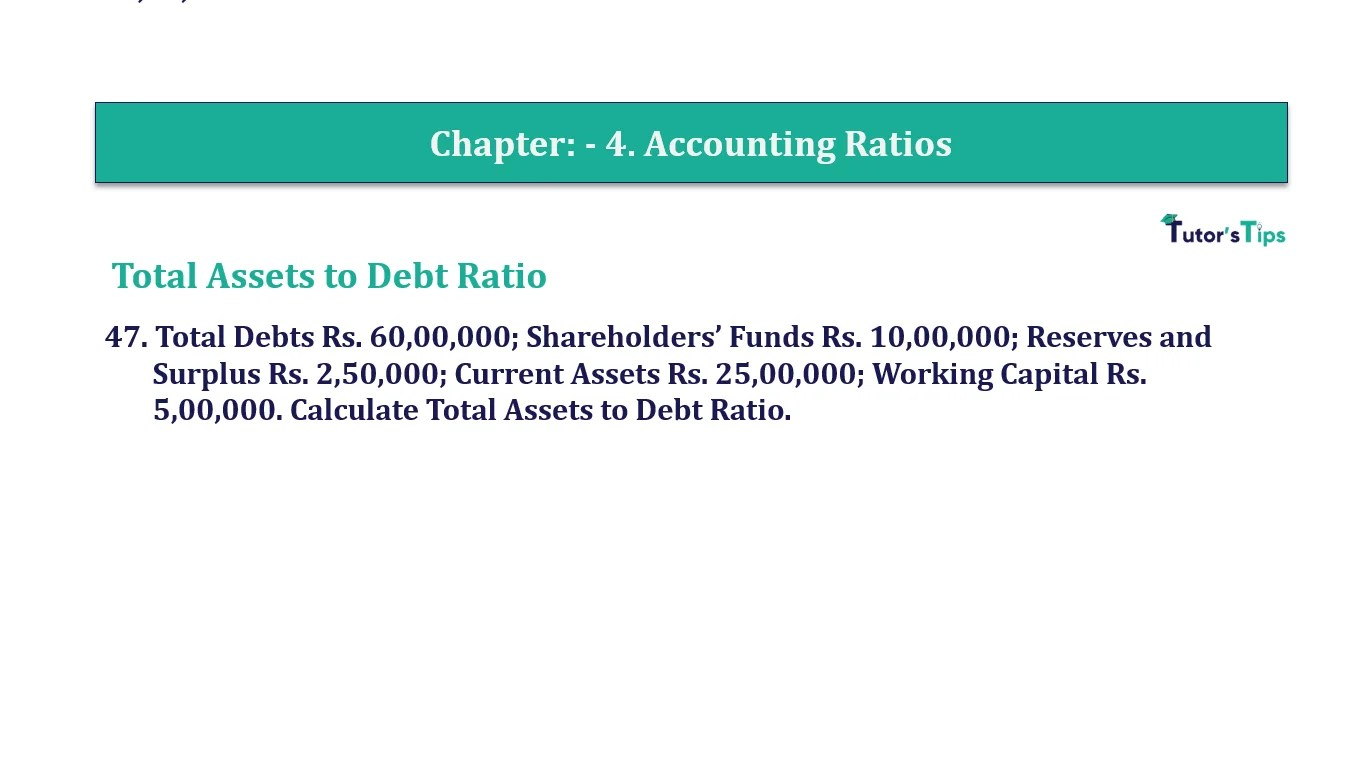# Question 47 Chapter 4 of +2-B – T.S. Grewal 12 ClassQuestion No. 47- Chapter No.4 - T.S. Grewal +2 Book Part B

Question 47 Chapter 4 of +2-B

Total Assets to Debt Ratio

47. Total Debts Rs. 60,00,000; Shareholders’ Funds Rs. 10,00,000; Reserves and Surplus Rs. 2,50,000; Current Assets Rs. 25,00,000; Working Capital Rs. 5,00,000. Calculate Total Assets to Debt Ratio.

### The solution of Question 47 Chapter 4 of +2-B: –

 Long-term Debts = Total Debts – Current Liabilities = Rs.60,00,000 – Rs.20,00,000 Long-term Debt = Rs. 40,00,000 Total Assets = Long-term Debts + Shareholders’ Funds + Current Liabilities = Rs.60,00,000 + Rs.10,00,000 Total Assets = Rs. 70,00,000

 Total Assets to Debt Ratio = Total Assets = Rs.70,00,000 Long- term Debts Rs.40,00,000 = 1.75: 1

Balance Sheet: Meaning, Format & Examples

Comment if you have any question.

Also, Check out the solved question of previous Chapters: –